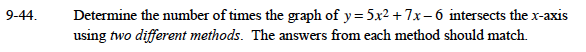### Home > CAAC > Chapter 9 > Lesson 9.2.2 > Problem9-44

9-44.Possible methods include substitution and graphing.

y = 5x2 + 7x − 6
y = 0

To use substitution set the two equations equal to each other.

5x2 + 7x − 6 = 0

Solve for x using the quadratic formula or by completing the square.

$x= \frac{-7±\sqrt{7^2 - 4(5)(-6)}}{(2)(5)}$